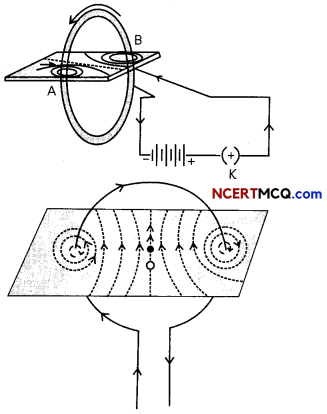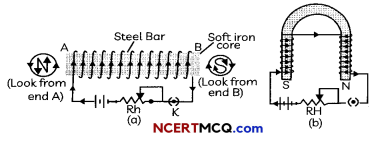## Magnetic Field Due to A Current Carrying Circular Wire

The magnetic field due to a current-carrying circular wire is shown in the figure below. Every section of the wire contributes to the magnetic field lines in the same direction within the loop.The field produced at the centre of a circular wire depends on the following factors:

• It is directly proportional to the strength of the current passing through it.
• It is inversely proportional to the radius of the loop.## Solenoid

A coil of many circular turns of wire wrapped in the shape of a cylinder, as shown in the Fig below is called a solenoid. The magnetic field, thus produced, is very much similar to that of a bar magnet. The field lines inside the solenoid are in the form of parallel straight lines. The magnetic field is the same at all points inside the solenoid. That is, inside the solenoid, the magnetic field is uniform.Magnetic Field of a Current-Carrying Solenoid Magnetic Field of a Bar Magnet 1. Solenoid is an electromagnet, i.e., the magnetic field will remain as long as current is passed through it. 1. Bar magnet is of permanent magnet. i.e.. there is no effect of current on its magnetic field. 2. The strength of the magnetic field of a solenoid depends on the number of turns of the coil ! and magnitude of electric current passed through it. 2. The strength of the magnetic field of a bar magnet cannot be changed. 3. The poles of the solenoid or the direction of magnetic field lines can be reversed by reversing the direction of current. 3. A bar magnet has fixed north and south poles which cannot be changed.

Electromagnets: When a material is placed inside a coil carrying current, it will get magnetised. A bunch of nails or an iron rod placed along the axis of the coil can be magnetized by the current passing through the coil. Once the current is switched-off the magnetic field will also be lost. Such magnets are called electro magnets.

The strength of an electromagnet depends upon the number of turns per unit length of the solenoid and the current through the solenoid.Example 1.
How does a solenoid behave like a magnet? Can you determine the north and south poles of a current-carrying solenoid with the help of a bar magnet? Explain.
Solenoid is a coil of many circular turns of insulated copper wire wrapped closely in the shape of a cylinder. A solenoid behaves like a magnet when current is passed through it. One end of the solenoid behaves as a magnetic north pole, while the other end behaves as the south pole.

We can use a bar magnet with known north poles near one end of the solenoid. If it shows repulsion then that end of solenoid is north pole and the other end is south pole. The property of magnet i.e. like poles repel and unlike poles attract is used for the determination of poles of solenoid.

Example 2.
List two methods of producing magnetic fields.